# High School Math : How to find the perimeter of a 45/45/90 right isosceles triangle

## Example Questions

### Example Question #4 : 45/45/90 Right Isosceles Triangles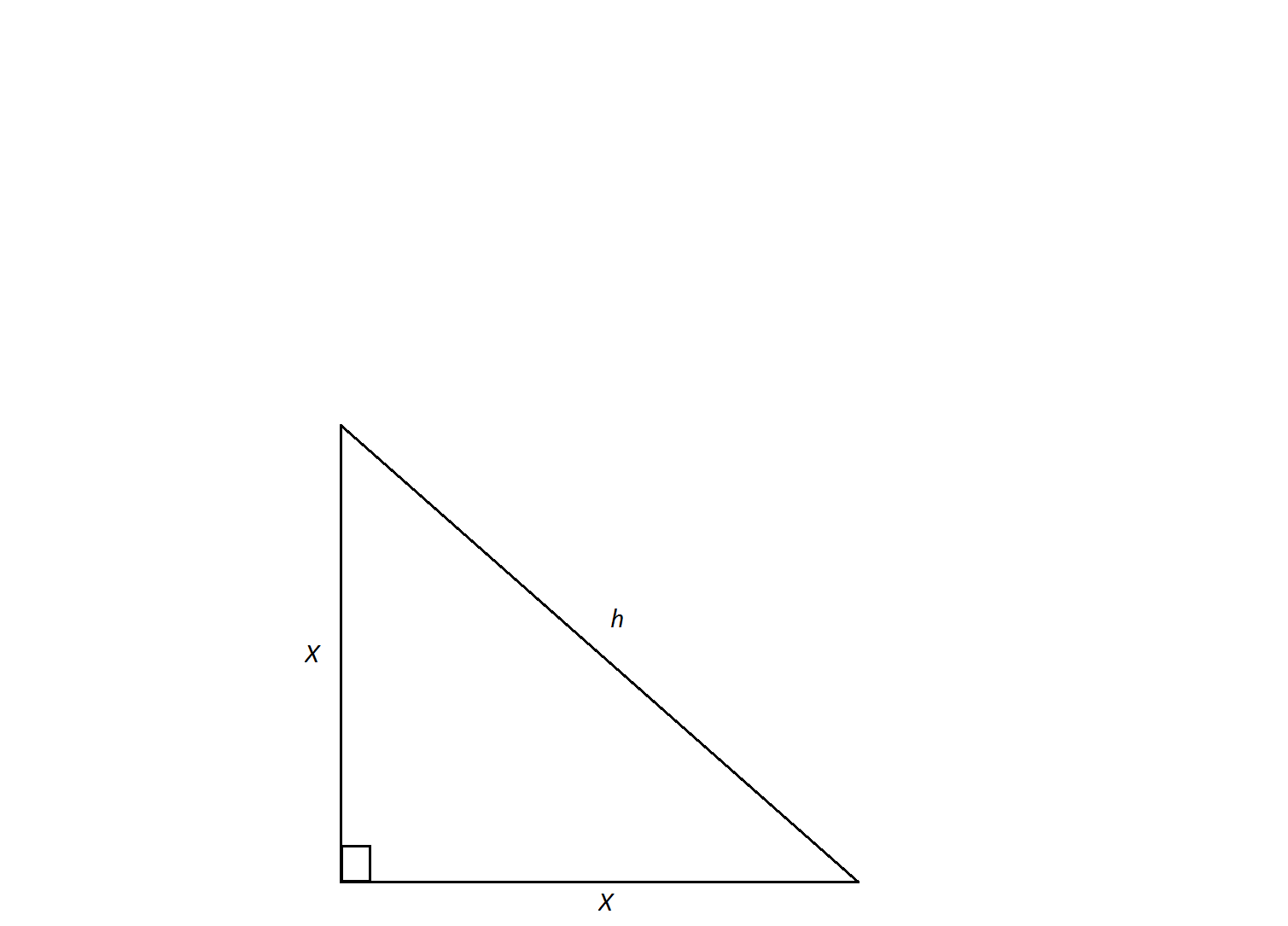The hypotenuse of an isosceles right triangle has a measure of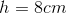. Find its perimeter.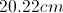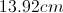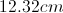Not enough information to solve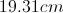Explanation:

In order to calculate the triangle's perimeter, we need to find the lengths of its legs.  An isosceles triangle is a special triangle due to the values of its angles. These triangles are referred to as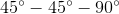triangles and their side lengths follow a specific pattern that states that one can calculate the length of the legs of an isoceles triangle by dividing the length of the hypotenuse by the square root of 2.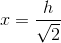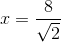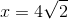Now we can calculate the perimeter by doublingand adding.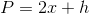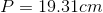### Example Question #5 : 45/45/90 Right Isosceles TrianglesThe side lengths of an isoceles right triangle measure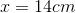. Find its perimeter.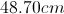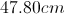Not enough information to solve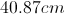Explanation:

An isosceles triangle is a special triangle due to the values of its angles.  These triangles are referred to astriangles and their side lenghts follow a specific pattern that states you can calculate the length of the hypotenuse of an isoceles triangle by multiplying the length of one of the legs by the square root of 2.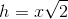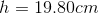Now we can calculate the perimeter by doublingand adding.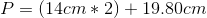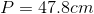### Example Question #6 : 45/45/90 Right Isosceles Triangles

A triangle has two angles equal to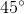and two sides equal to. What is the perimeter of this triangle?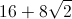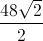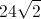Explanation:

When a triangle has two angles equal to, it must be a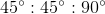isosceles right triangle.

The pattern for the sides of ais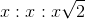.

Since two sides are equal to, this triangle will have sides of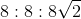.

Add them all together to get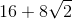.

### Example Question #7 : 45/45/90 Right Isosceles Triangles

An isosceles triangle has a base of 6 and a height of 4. What is the perimeter of the triangle?None of these